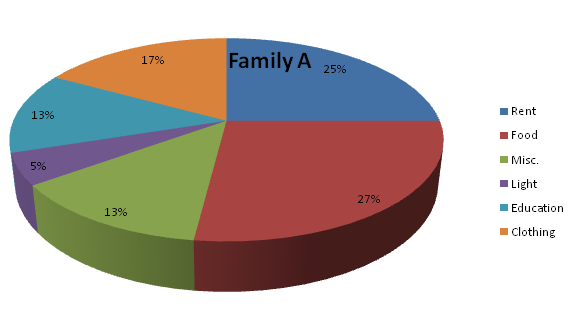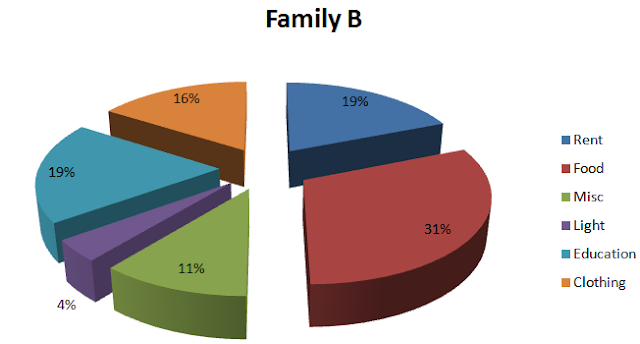# Data Interpretation - IBPS Clerk Set

Directions for questions 1 - 6: Refer to the following pie charts and solve the questions based on it.Total Expenses = Rs. 48, 000Total Expenses = Rs. 72,000
Q1. Both the families decide to double the total expenditure keeping the pattern of spending the same as given above. What will be the new ratio of expenditure on food between family A and family B?
a) 18 : 31
b) 31 : 27
c) 2 : 3
d) 3 : 2
e) None of The Above

Q2. If the total expenses of family B increases three - fold, keeping the expenses on education the same as given above, what will be the expense on education?
a) 6.33%
b) 57%
c) 19%
d) 18%
e) None of The Above

Q3. What will be the expenses on light by family A, as a percentage of expense on light by family B?
a) 120%
b) 83.33%
c) 62.5%
d) 66.66%
e) None of The Above

Q4. If family A and family B decide to combine their expenses, then which one of the following heads will be responsible for the highest expenses?
a) Rent
b) Miscellaneous
c) Food
d) Education
e) None of The Above

Q5. In the above question, how many heads will have a lower percentage share in the combined total expenses of both the families than the percentage share of family B under the same head?
a) 1
b) 2
c) 3
d) 4
e) None of The Above

Q6. Under how many heads are the expenses of family B more than the expenses of family A?
a) Less than 3
b) More than 3
c) Equal to 3
d) Can't be Determined
e) None of The Above

### Solution

If the percentage increase in the expenditure of both the families, is the same then the ratio will be the same.

The total consumption has become 3 times more keeping the expenses on education the same. Hence, the percentage consumption on education will become 1/3rd of the person

By visual inspection we can see that option C is the correct answer

It should be understood that the final percentage of expenditure will always be in between the percentage of family A and family B (it is true of any mixture that the percentage composition of the mixture will be always in between the percentage compositions of  the components).

By visual inspection we can see the correct answer is option B.

==>> Follow Data Interpretation Guide (50 sets + Video lecture)